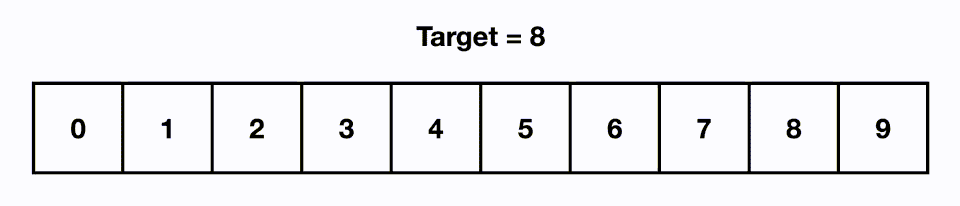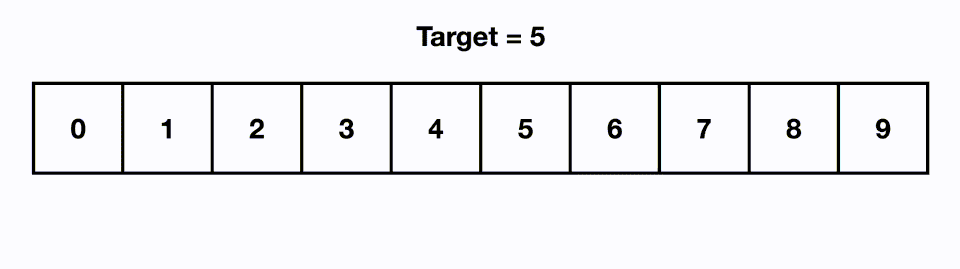April 23, 2023 • 11 min read

# Linear & Binary Search

AuthorTunar
@Tunar

Tags

#Computer Science#Datastrucutre#Binary Search#Linear Search#Algorithms

Next ArticleSearch algorithms are one of the most important topics in computer science. In this article, we will take a look at binary and linear search algorithms. Search algorithms are used to search for a specific data in a data structure. Different search algorithms are used in different situations. First, let's take a look at the linear search algorithm.

### Linear Search

The Linear Search algorithm is a simple algorithm used to search for a given data in a given dataset. Its time complexity is O(n), meaning that in the worst case scenario, it searches through all the data in the dataset one by one.

The working principle of the algorithm is quite simple. Each element in the given dataset is compared with the searched value. If the searched value matches an element, the index of that element is returned. If there is no matching element, -1 is returned.

The Linear Search algorithm can work quite fast on small datasets. However, it is a slow algorithm for large datasets. Therefore, more efficient algorithms should be preferred for large datasets.```cpp```    int linearSearch(int arr[], int n, int x) {
for (int i = 0; i < n; i++) {
if (arr[i] == x) {
return i;
}
}
return -1;
}``````

### Binary Search

Actually, since this article is more focused on binary search, more examples and information will be provided here. To use the binary search algorithm, your dataset must be sorted. Otherwise, it will not work correctly. The binary search algorithm is better than the linear search algorithm in terms of time complexity. The time complexity of the binary search algorithm is O(log N). This is because the binary search algorithm divides the dataset in half every time it cannot find the required data. You can see this better in the graph below. The binary search algorithm looks at the middle element each time. If the element is equal to the searched value, the process ends and the data is found. Otherwise, the algorithm checks whether the middle element in the interval is greater or less than the searched value. Based on this, the decision is made on which interval to search for the data in the next step. If the element is greater, we know that the searched value is to the left of the current element, or if the element is smaller, we know that the searched value is to the right of the element. These operations continue until there is no interval left to search, and if the data has not been found yet, we can say that the data is not in the dataset. That's why we need a sorted dataset.```cpp```    int binarySearch(int arr[], int n, int x) {
int left = 0, right = n - 1;
while (left <= right) {
int mid = (left + right) / 2;
if (arr[mid] == x) {
return mid;
}
if (arr[mid] < x) {
left = mid + 1;
}
else {
right = mid - 1;
}
}
return -1;
}``````

### Example questions 1.

We can quickly find the k-th digit of 2, 3, or 5 in the range [1, 10^5] using the binary search algorithm. But first, let's see how we can check for it. It requires some mathematical knowledge, and we can use the Inclusion-Exclusion Principle. I recommend doing some research to understand this topic. Now, let's create a formula.

1. The number of digits divisible by 2 in the range [1, N] is N/2.
2. The number of digits divisible by 3 in the range [1, N] is N/3.
3. The number of digits divisible by 5 in the range [1, N] is N/5.

Now let's add them, but it's not over yet because there are digits that can be divisible by 2, 3, and 5 at the same time. For example, the number 30 can be divisible by 2, 3, and 5. Therefore, our formula is not complete yet.

1. The number of digits divisible by both 2 and 3 in the range [1, N] is N/6.
2. The number of digits divisible by both 2 and 5 in the range [1, N] is N/10.
3. The number of digits divisible by both 3 and 5 in the range [1, N] is N/15.

Now let's subtract them, but the formula is still incomplete. Finally, let's add N/30, and our formula for the number of digits divisible by 2, 3, and 5 in the range [1, N] is N/2 + N/3 + N/5 — N/6 — N/10 — N/15 + N/30. Now let's write our code.

```cpp```    int binarySearch(int k) {
int left = 1, right = 1e5, mid;
while (right >= left) {
mid = (left + right) / 2;
int count = mid / 2 + mid / 3 + mid / 5 - mid / 6 - mid / 10 - mid / 15 + mid / 30;

if (count == k) {
break;
}
if (count > k) {
right = mid - 1;
}
else {
left = mid + 1;
}
}

if (mid % 2 != 0 && mid % 3 != 0 && mid % 5 != 0) {
mid -= min({mid % 2, mid % 3, mid % 5});
}

return mid;
}``````

I think this problem is a good example because now we understand how to use the binary search algorithm with other data structures. In this problem, we used integers as the data set.

### Example questions 2.

We need to divide N digits in an array into K different groups, such that the sum of digits in the largest group is minimized. For example, let N = 5, K = 3, and A = [2, 4, 7, 3, 5]. This array can be divided into 3 different groups as [2, 4], , [3, 5], and this is the minimum sum of maximum sums of subarrays. To solve this problem, we can perform a search in the range [1, 10¹⁸]. In this problem, we are looking for the minimum sum, so we will use binary search to approach the answer gradually. I know that this question may be a bit difficult for beginners in binary search, but I will try to explain it well. Let our control method be as follows:

```cpp```    bool check(int n, int k, int arr[], long long mid) {
int count = 0;
long long sum = 0;
for (int i = 0; i < n; ++i) {
if (arr[i] > mid) return false;
if (sum + arr[i] > mid) {
count++;
sum = 0;
}
sum += arr[i];
}
if (sum > 0) count++;
return count <= k;
}``````

We are checking whether the mid sum is possible in the given array. While traversing the array, we do the following:

1. If any number in the array is greater than mid, then it is impossible to form this sum.

2. While traversing the array, if the next number is greater than mid minus the current sum, then it means we have formed a group and need to form a new group. Therefore, we set sum = 0 and increment count.

3. We add new numbers to the sum.

If there is any remaining sum, then it means a new group is formed. Therefore, we increment the group and can return whether the mid sum is possible as a result. So, our code looks like this:

```cpp```    long long binarySearch(int n, int k, int arr[]) {
long long left = 1, right = 1e18, answer;
while (right >= left) {
long long mid = (left + right) / 2;
if (check(n, k, arr, mid)) {
right = mid - 1;
} else {
left = mid + 1;
}
}
}``````

As you can see in this problem, since we don't know the answer beforehand, we try to approach the closest possible answer step by step. Therefore, we set mid as the answer.

I hope it has been useful for everyone. I tried to explain it as well as possible.Void Development

Void Development is a development team founded in 2019. We generally support open-source projects. And of course, we are always developing new projects.